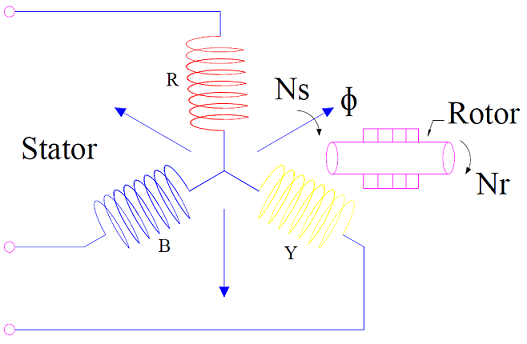What is Slip in Induction Motor?

Slip in Induction Motor is the relative speed between the rotating magnetic flux and rotor expressed in terms of per unit synchronous speed. It is a dimensionless quantity. The value of slip in induction motor is can never be zero.

If Ns and Nr being the synchronous speed of rotating magnetic flux and rotor speed respectively, then the relative speed between them is equal to (Ns – Nr). Therefore, slip is defined as

Slip (s) = (Ns – Nr) / Ns

As we know that, the speed of rotor cannot be equal to synchronous speed i.e. Nr < Ns, the value of slip s is always less than one. For induction motor, 0<s<1.

When a three phase power supply is connected to the three phase stator winding of induction motor, a rotating magnetic field is produced in the air gap. The speed of this rotating magnetic field is called the synchronous speed. Synchronous speed is determined by the number of poles (P) and frequency (f) of power supply. Synchronous speed is equal to N = 2f / P rps (revolution per second).This rotating magnetic field cuts the stationary rotor conductors to generate emf. As rotor circuit is short circuited, this generated emf gives rise to rotor current. The interaction of this rotor current with rotating magnetic flux produces a torque and hence the rotor begins to rotate in the direction of rotating magnetic field as per lenz’s law. Consequently a relative speed equal to (Ns – Nr) is set up between them and gives rise to slip in induction motor.

Why Slip is Never Zero in an Induction Motor?

Zero slip means that rotor speed is equal to synchronous speed. If rotor is rotating at synchronous speed in the direction of rotating magnetic field the, there will be no flux cutting action, no emf in the rotor conductors, no current in rotor bar conductor and hence no development of electromagnetic torque. Thus rotor of 3 phase Induction Motor can never attain synchronous speed. Therefore, slip is never zero in induction motor.

Different Values of Slip and Significance:

The significance of slip in induction machine is tabulated below. The behavior of induction machine is quite dependent on the value of slip.

 Value of Slip (s) Significance s = 0 (Zero Slip) Zero slip means that rotor speed is equal to synchronously rotating magnetic flux. Under this condition, there will not be any relative motion between the rotor coils and rotating magnetic flux. Therefore, there will not be any flux cutting action of rotor coils. Hence, no emf will be generated in rotor coils to produce rotor current. This means no electromagnetic torque will be produced. Induction motor will not work. Therefore, it very important for induction motor to have a positive value of slip. This is the reason; slip is never zero in an induction motor. s  = 1 (Slip equal to 1) Slip = 1, means that rotor is stationary. s = Negative (Negative Slip) Negative value of slip in induction motor can be achieved when the rotor speed is more than the synchronously rotating magnetic flux. This is only possible, when the rotor is rotated in the direction of rotating magnetic flux by some prime mover. Under this condition, the machine operates as an Induction Generator. Read Torque Slip Characteristics for detail. s > 1 (Slip greater than 1) Slip more than 1 implies that, rotor is rotating in a direction opposite to the direction of rotation of magnetic flux. This means if magnetic flux is rotating in clockwise direction, then rotor is rotating in anticlockwise direction or vice versa. Therefore, the relative speed between them will be (Ns + Nr). In Plugging or Braking of Induction motor, slip more than 1 is achieved to quickly bring the rotor at rest.

This site uses Akismet to reduce spam. Learn how your comment data is processed.